Next: Probability and independence Up: TIME-STATISTICAL RESOLUTION Previous: Ensemble

## Expectation and variance

A conceptual average over the ensemble, or expectation, is denoted by the symbol.The index for summation over the ensemble is never shown explicitly; every random variable is presumed to have one. Thus, the true mean at time t is defined as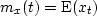.The mean can vary with time: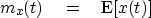(12)
The variance''is defined to be the power after the mean is removed, i.e.,(13)
(Conventionally,is referred to as the variance, andis called the standard deviation.'')

For notational convenience, it is customary to write m(t),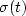, and x(t) simply as m,, and xt, using the verbal context to specify whether m andare time-variable or constant. For example, the standard deviation of the seismic amplitudes on a seismic trace before correction of spherical divergence decreases with time, since these amplitudes are expected to be globally'' smaller as time goes on.

When manipulating algebraic expressions, remember that the symbolbehaves like a summation sign, namely,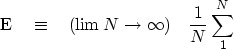(14)
Note that the summation index is not given, since the sum is over the ensemble, not time. To get some practice with the expectation symbol, we can reduce equation (13):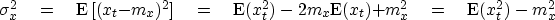(15)
Equation (15) says that the energy is the variance plus the squared mean.Next: Probability and independence Up: TIME-STATISTICAL RESOLUTION Previous: Ensemble
Stanford Exploration Project
10/21/1998